Open Logic Project Builds Site

# Open Logic Project Builds

This site contains PDFs built from the source LaTeX files of the most recent version of the Open Logic Text.

## Complete PDFs

We have PDFs of the complete text in the Open Logic `master` branch, arranged in a somewhat sensible manner, including editorial comments. It's not intended as a textbook, but it shows what's there.

## Individual Textbooks

There are already a few textbooks that show how one might "remix" the material in the Open Logic Project to produce nice textbooks. These vary in the material that's included, the design, and the configuration options used. They may also include additional material specific to those courses.

### Sets, Logic, Computation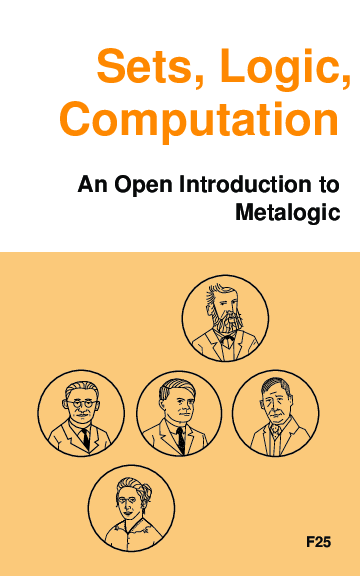A textbook on metalogic, developed for Calgary's Logic II course: includes the material on set theory, first-order logic (including both sequent calculus and natural deduction), and Turing machines (including undecidability). Appendices cover proofs and induction and biographies of some logicians. This text also includes chapter summaries and a glossary. Problems are collected at the end of chapters.

• slc-screen.pdf for screen reading (color, smaller margins, low-res images)
• slc-print.pdf for print-on-demand (without cover)
• Source code for generating this textbook is on GitHub in a separate repository.

### Incompleteness and Computability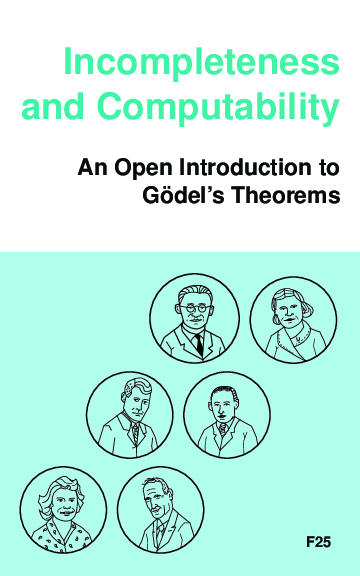A textbook on Gödel's incompleteness theorems and computability theory, developed for Calgary's Logic III course: includes the material on recursive functions, Gödel's incompleteness theorem, models of arithmetic, second-order logic, and the lambda calculus. Appendices cover basics of first-order logic with natural deduction, and biographies of some logicians. This text also includes chapter summaries.

• ic-screen.pdf for screen reading (color, smaller margins, low-res images)
• ic-print.pdf for print-on-demand (without cover)
• Source code for generating this textbook is on GitHub in a separate repository.

### Boxes and Diamonds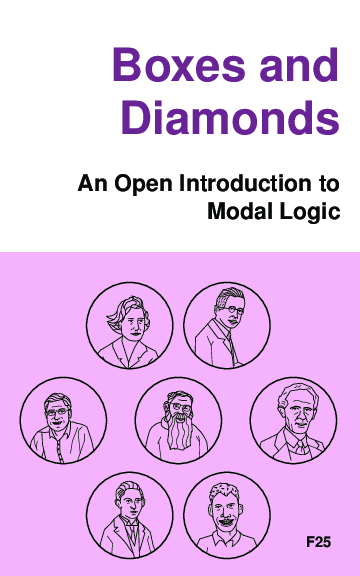A textbook for modal and other intensional logics based on the Open Logic Project; includes the material on normal modal logic, intutionistic logic, and counterfactuals, with appendices on basic set theory and classical propositional logic.

• bd-screen.pdf for screen reading (color, smaller margins, low-res images)
• Source code for generating this textbook is on GitHub in a separate repository.

### Set Theory: An Open IntroductionA brief introduction to the philosophy of set theory; covers why set theory came about; how to reduce large swathes of mathematics to set theory + arithmetic; how to embed arithmetic in set theory; what the cumulative iterative conception of set amounts to; how one might try to justify the axioms of ZFC. Most of the material making up the text comes from Tim Button's Open Set Theory.

• settheory-screen.pdf for screen reading (color, smaller margins, low-res images)
• Source code for generating this textbook is on GitHub in a separate repository.

### Intermediate Logic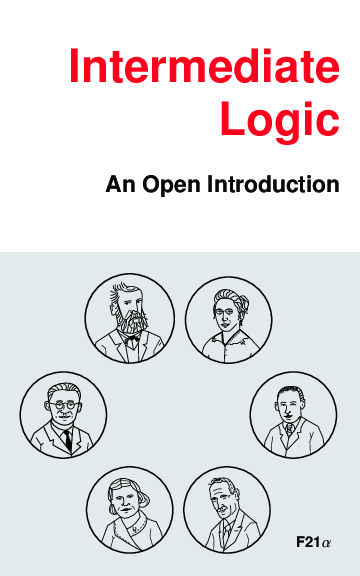A textbook for McGill's Intermediate Logic (Phil 310) course: includes the material on naïve set theory, first order logic through the Completeness Theorem, recursive functions, Gödel's incompleteness theorem, and models of arithmetic. Appendices cover proofs and induction and biographies of some logicians. This text also includes chapter summaries.

• il-screen.pdf for screen reading (color, smaller margins, low-res images)
• Source code for generating this textbook is on GitHub in a separate repository.

### What If?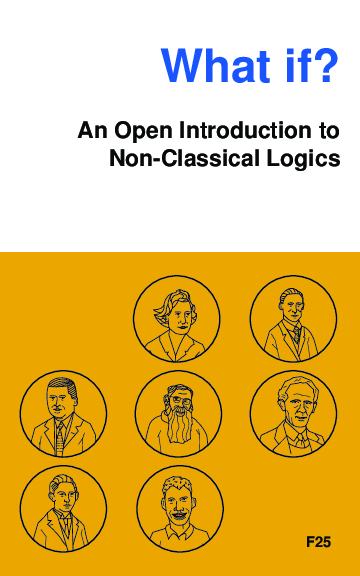A textbook used for Calgary's Philosophy of Logic (Phil 473) and Victoria's Non-Classical Logics (Phil 371) courses. It includes the OLP material on propositional classical, many-valued, modal (including epistemic, temporal, and conditional logics), and intuitionistic logics. Appendices cover sets and relations, as well as proofs and induction. This is currently an incomplete draft. Material on epistemic and temporal logic is yet to be merged into the main OLP repository.

• wi-screen.pdf for screen reading (color, smaller margins, low-res images)
• Source code for generating this textbook is on GitHub in a separate repository.

### Sample Texts

The OLP main repository also includes two sample textbooks that serve just to illustrate how one might produce texts using different formatting conventions.

• open-logic-enderton, a sample textbook that follows the conventions of Enderton's popular A Mathematical Introduction to Logic.
• open-logic-sample, a selection including set theory, first-order logic, Turing machines, and incompleteness, using yet other conventions.

## Active Branches

At any point, there may be active branches of the Open Logic Project in GitHub. This branches may contain additional material, or revisions of the material in the `master` branch which are being worked on or reviewed for inclusion. We provide PDFs of the complete text as it looks in these branches.